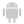Home |Sign in | EnglishHelpWLanguageWLanguage functionsControls, pages and windowsDrawing functionsPrefix syntaxDrawing functions on WDPic variablesPicRotationPresentationExampleRelated examplesSee alsoOpenSaveAlphaBlendBackgroundChangeModeDrawArcDrawChordDrawCircleDrawLineDrawPointDrawPolygonDrawPolylineDrawRectangleDrawRectangleGradientDrawRoundedRectangleDrawSliceDrawTextDrawTextRTFEndDrawingFillFontHorizontalSymmetryInvertColorMergeLayerPixelColorPixelOpacityResizeRotationSaveStartDrawingVerticalSymmetryPicMergeLayerPicOpenPicResizePicRotationPicSave
WINDEVWEBDEVWINDEV MobileOthersPicRotation (Function)
In french: PicRotation
Rotates a WDPic image. All image layers rotate.
Remark: To rotate one single layer, use dRotation.
Example
// Open a source image
MyWDPicImage is WDPic
MyWDPicImage.FileName = fExeDir() + ["\"] + "Source.png"

// Rotate the image
PicRotation(MyWDPicImage, 45)

// Save the result
PicSave(MyWDPicImage, fExeDir() + ["\"] + "Result.png")
Syntax

Performing a simple rotation

<Result> = PicRotation(<WDPic image> , <Angle> [, <Options>])
<Result>: Boolean
• True if the rotation was performed,
• False otherwise.
<WDPic image>: WDPic variable
Name of the WDPic variable that contains the image to use.
<Angle>: Real
Rotation angle (in degrees).
• If the angle is positive, the image rotates clockwise.
• If the angle is negative, the image rotates counterclockwise.
<Options>: Integer constant
Rotation options:
 drAdapt The image is flipped and enlarged. Then, its size is reduced to correspond to the initial image size. drDefault(default value) Enlarge the image (if necessary) so that it corresponds to the dimensions of rotated image. drNoEnlarging The image is turned but it is not enlarged: the image may be truncated.

Performing a rotation while modifying the rotation center

<Result> = PicRotation(<WDPic image> , <Angle> , <X> , <Y> [, <Options>])
<Result>: Boolean
• True if the rotation was performed,
• False otherwise.
<WDPic image>: WDPic variable
Name of the WDPic variable that contains the image to use.
<Angle>: Real
Rotation angle (in degrees).
• If the angle is positive, the image rotates clockwise.
• If the angle is negative, the image rotates counterclockwise.
<X>: Real
New X-coordinate of rotation center.
<Y>: Real
New Y-coordinate of rotation center.
<Options>: Optional Integer constant
Rotation options:
 drDefault(Default value) Enlarge the image (if necessary) so that it corresponds to the dimensions of rotated image. drNoEnlarging The image is flipped but not enlarged
Related Examples:Unit examples (WINDEV): WDPic type [ + ] This example shows how to use the WDPic WLanguage type.
Component: wd270pic.dll
Minimum version required
• Version 25